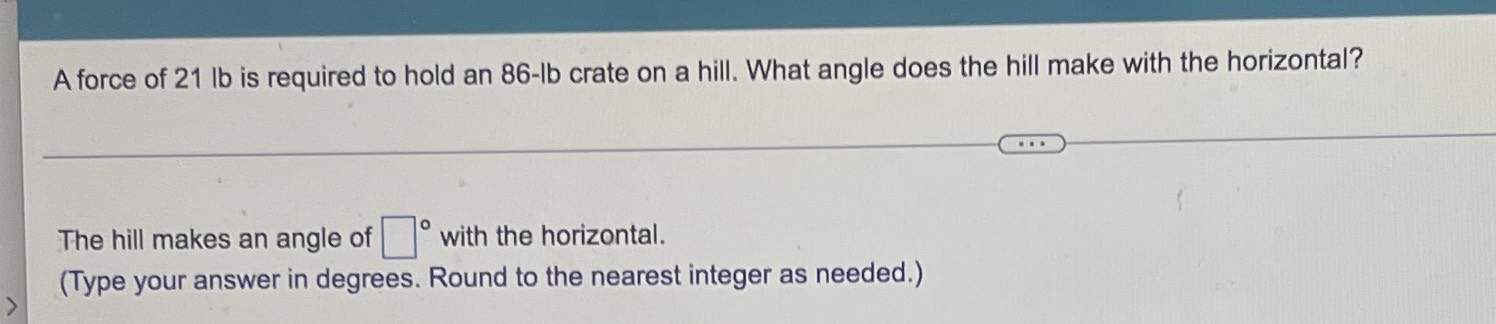### Still have math questions?A force of $$21 lb$$ is required to hold an $$86 - lb$$ crate on a hill. What angle does the hill make with the horizontal? The hill makes an angle of $$\square ^ { \circ }$$ with the horizontal. (Type your answer in degrees. Round to the nearest integer as needed.)